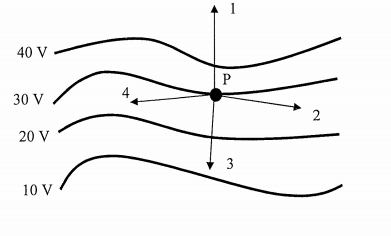# Problem: For the equipotential lines shown in the diagram, the vector that best represents the direction of the electric field a point "P" is: A) Vector 1 B) Vector 2 C) Vector 3 D) Vector 4

###### FREE Expert Solution
87% (379 ratings)
###### Problem Details

For the equipotential lines shown in the diagram, the vector that best represents the direction of the electric field a point "P" is:

A) Vector 1

B) Vector 2

C) Vector 3

D) Vector 4Frequently Asked Questions

What scientific concept do you need to know in order to solve this problem?

Our tutors have indicated that to solve this problem you will need to apply the Equipotential Surfaces concept. You can view video lessons to learn Equipotential Surfaces. Or if you need more Equipotential Surfaces practice, you can also practice Equipotential Surfaces practice problems.

How long does this problem take to solve?

Our expert Physics tutor, Juan took 1 minute and 43 seconds to solve this problem. You can follow their steps in the video explanation above.

What professor is this problem relevant for?

Based on our data, we think this problem is relevant for Professor Stoneback's class at UTD.